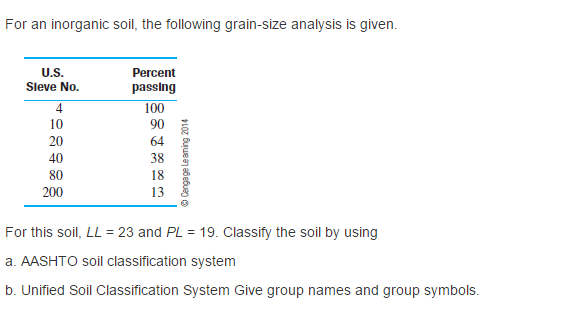# Question For an inorganic soil, the following grain-size analysis is given. For this soil, LL = 23 and PL = 19. Classify the soil by using a. AASHTO soil classification system b. Unified Soil Classification System Give group names and group symbols.PCORJC The Asker · Civil EngineeringTranscribed Image Text: For an inorganic soil, the following grain-size analysis is given. For this soil, LL = 23 and PL = 19. Classify the soil by using a. AASHTO soil classification system b. Unified Soil Classification System Give group names and group symbols.
More
Transcribed Image Text: For an inorganic soil, the following grain-size analysis is given. For this soil, LL = 23 and PL = 19. Classify the soil by using a. AASHTO soil classification system b. Unified Soil Classification System Give group names and group symbols.